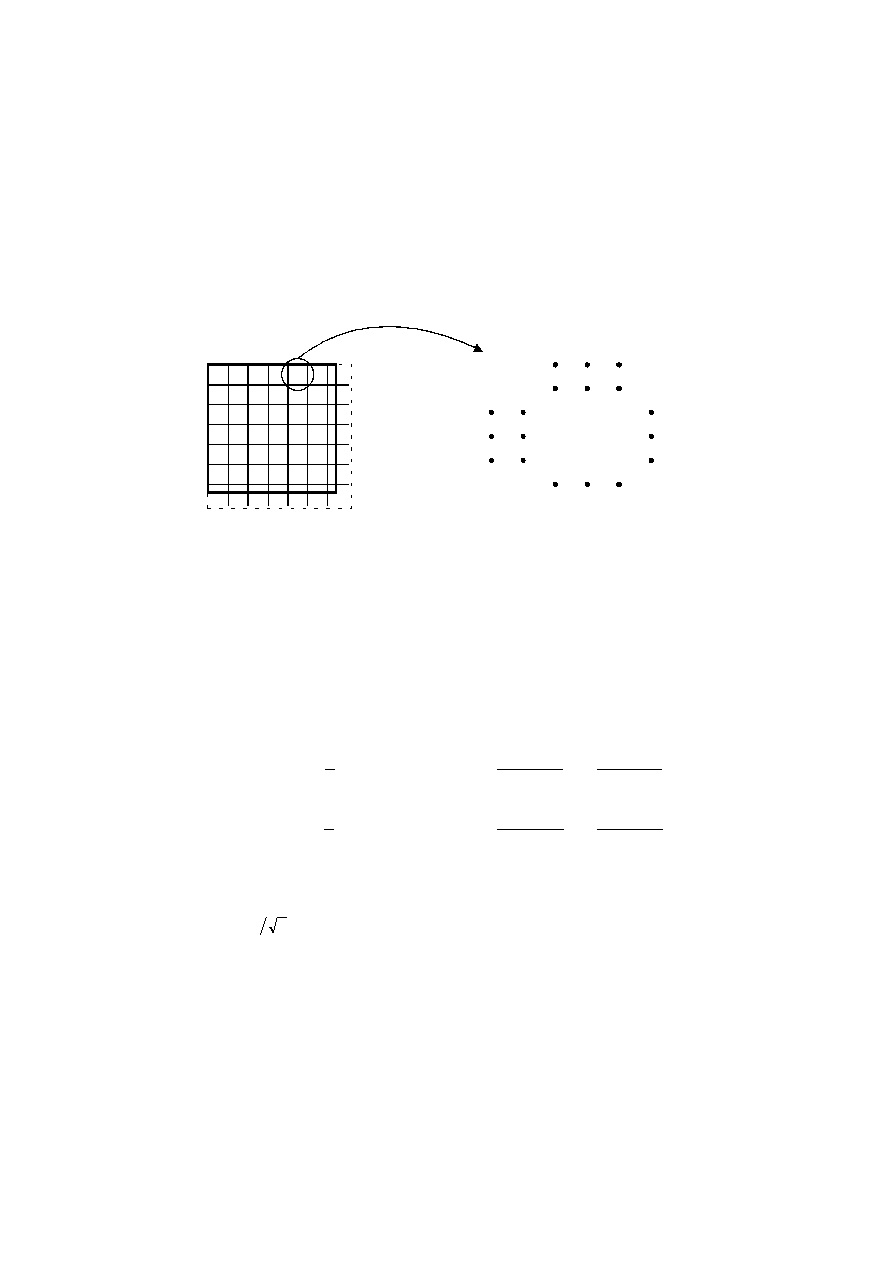ISO/IEC 10918-1 : 1993(E)
After a non-differential frame decoding process computes the IDCT and produces a block of reconstructed image samples,
an inverse level shift shall restore the samples to the unsigned representation by adding 2
P ­ 1
and clamping the results to
the range 0 to 2
P ­ 1
.
A.3.2
Orientation of samples for FDCT computation
Figure A.4 shows an image component which has been partitioned into
8
×
8
blocks for the FDCT computations. Figure
A.4 also defines the orientation of the samples within a block by showing the indices used in the FDCT equation of A.3.3.
The definitions of block partitioning and sample orientation also apply to any DCT decoding process and the output
reconstructed image. Any sample added by an encoding process to complete partial MCUs shall be removed by the
decoding process.
C
i
00
s
s
s
s
s
s
s
s
s
01
10
11
70
71
77
17
07
TISO0810-93/d017
Top
Left
Right
Bottom
Figure A.4 ­ Partition and orientation of 8 x 8 sample blocks
FIGURE A.4 [D17] 6 cm = 234 %
A.3.3
FDCT and IDCT (informative)
The following equations specify the ideal functional definition of the FDCT and the IDCT.
NOTE ­ These equations contain terms which cannot be represented with perfect accuracy by any real implementation. The
accuracy requirements for the combined FDCT and quantization procedures are specified in Part 2 of this Specification. The accuracy
requirements for the combined dequantization and IDCT procedures are also specified in Part 2 of this Specification.
FDCT:
IDCT:
S
C
C
s
x
u
y
v
s
C C
S
x
u
y
v
vu
u
v
x
y
yx
yx
u
v
u
v
vu
=
+
+
=
+
+
=
=
=
=
1
4
2
1
16
2
1
16
1
4
2
1
16
2
1
16
0
7
0
7
0
7
0
7
cos
(
)
cos
(
)
cos
(
)
cos
(
)
where
C
C
u v
u
v
,
=
=
1
2
0
for
,
C
C
u
v
,
=
1 otherwise
otherwise.
A.3.4
DCT coefficient quantization
(informative) and dequantization (normative)
After the FDCT is computed for a block, each of the 64 resulting DCT coefficients is quantized by a uniform quantizer.
The quantizer step size for each coefficient S
vu
is the value of the corresponding element Q
vu
from the quantization table
specified by the frame parameter Tq
i
(see B.2.2).
CCITT Rec. T.81 (1992 E)
27